We think you are located in United States. Is this correct?

# End of chapter exercises

## End of chapter exercises

Textbook Exercise 7.6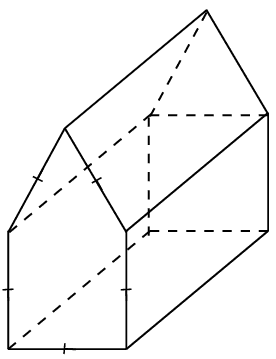Describe this figure in terms of a prism.
Square prism with a triagonal prism on one side. Note that this is not a pentagonal prism since not all the angles are the same size.
Draw a net of this figure.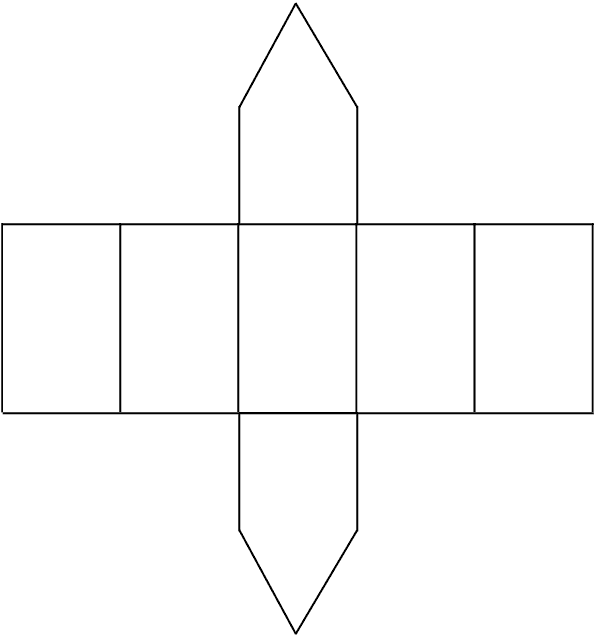Which of the following is a net of a cube?

1.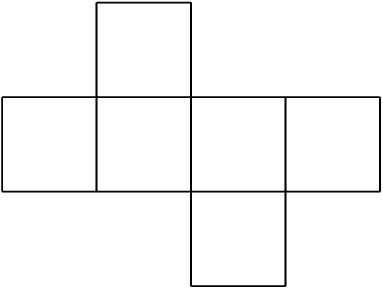2.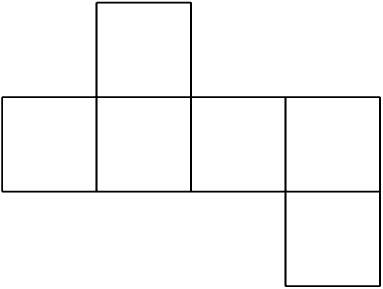3.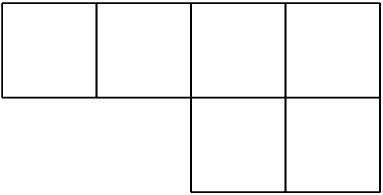4.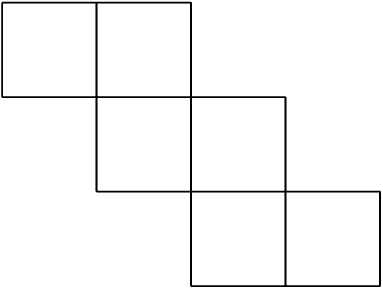5.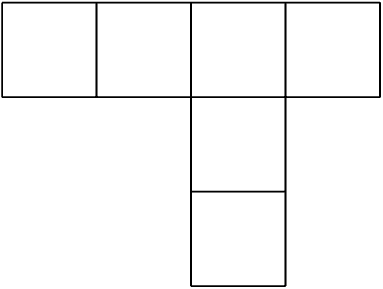a and d

Name and draw the following figures:

A prism with the least number of sides.
Triangular prism
A pyramid with the least number of vertices.
Triangular pyramid
A right prism with a kite base.
Rhombic prism

Determine how much paper is needed to make a box of width $$\text{16}$$ $$\text{cm}$$, height $$\text{3}$$ $$\text{cm}$$ and length $$\text{20}$$ $$\text{cm}$$ (assume no overlapping at corners).

\begin{align*} \text{Box surface area}&=2[3\times16+3\times20+16\times20] \\ &=2 \\ &= \text{856}\text{ cm$^{2}$} \end{align*}
Give a mathematical name for the shape of the box.
Rectangular prism
Calculate the volume of the box.
\begin{align*} \text{Volume } &=3\times16\times20 \\ &=\text{960}\text{ cm$^{3}$} \end{align*}

Determine how much paper is needed to make a cube with a capacity of $$\text{1}$$ $$\text{L}$$.

\begin{align*} 1 \text{ L}&= \text{1 000}\text{ cm$^{3}$} \\ \therefore \text{Side length }&= \text{10}\text{ cm} \\ \text{Surface area}&=6\times(10\times10) \\ &= \text{600}\text{ cm$^{2}$} \end{align*}

Compare the box and the cube. Which has the greater volume and which requires the most paper to make?

The cube has a greater volume and the box requires more paper to make.

$$ABCD$$ is a rhombus with sides of length $$\frac{3}{2}x$$ millimetres. The diagonals intersect at $$O$$ and length $$DO = x$$ millimetres. Express the area of $$ABCD$$ in terms of $$x$$.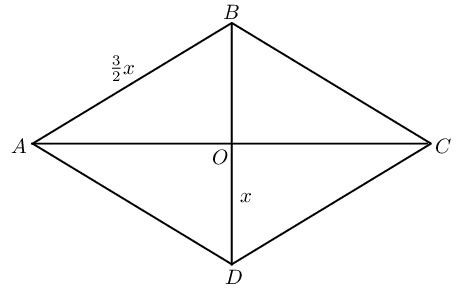\begin{align*} AD &= \frac{3}{2}x \\ DO&=x \\ AO^2&=\left ( \frac{3}{2}x \right )^2-x^2 \quad (\text{ Pythagoras})\\ &=\frac{9}{4}x^2-x^2\\ &=\frac{5}{4}x^2 \\ \therefore AO&=\frac{x\sqrt{5}}{2} \\ \therefore AC&=x\sqrt{5} \\ \text{Area }&=\frac{1}{2}AC\times BD\\ &=\frac{1}{2}\times x \sqrt{5} \times 2x \\ &=\sqrt{5} x^2 \end{align*}

The diagram shows a rectangular pyramid with a base of length $$\text{80}$$ $$\text{cm}$$ and breadth $$\text{60}$$ $$\text{cm}$$. The vertical height of the pyramid is $$\text{45}$$ $$\text{cm}$$.Calculate the volume of the pyramid.
\begin{align*} \text{Volume }&=\frac{1}{3}\times\text{area of base}\times\text{height} \\ &=\frac{1}{3}(80\times60)\times45 \\ &=\text{72 000}\text{ cm$^{3}$} \end{align*}
Calculate $$H$$ and $$h$$.
\begin{align*} H^2&=30^2+45^2 \quad (\text{ Pythagoras})\\ &= \text{2 925} \\ \therefore H&=\text{54}\text{ cm} \\ h^2&=45^2+40^2 \quad (\text{ Pythagoras})\\ h&=\text{60,2}\text{ cm} \end{align*}
Calculate the surface area of the pyramid.
\begin{align*} \text{Surface area }&=2 \left (\frac{1}{2}\times80\times H \right )+2\left ( \frac{1}{2} \times 60\times h\right )+80\times 60\\ &=(80 \times 54)+(60 \times \text{60,2})+\text{4 800}\\ &=\text{12 732}\text{ cm$^{2}$} \end{align*}

A group of children are playing soccer in a field. The soccer ball has a capacity of $$\text{5 000}$$ $$\text{cc}$$ (cubic centimetres). A drain pipe in the corner of the field has a diameter of $$\text{20}$$ $$\text{cm}$$. Is it possible for the children to lose their ball down the pipe? Show your calculations.

\begin{align*} \text{Volume of ball }&= \frac{4}{3}\pi r^3 \\ &=\text{5 000}\\ \therefore r&= \text{10,6} \\ \text{And diameter }&=\text{21,2}\text{ cm}\\ \text{Diameter of pipe}&=\text{20}\text{ cm} \end{align*}

No, the ball is too big to go down the drain pipe.

A litre of washing powder goes into a standard cubic container at the factory.

Determine the length of the sides of the container.
$\text{Dimensions: } \text{10}\text{ cm}\times\text{10}\text{ cm}\times\text{10}\text{ cm}$
Determine the dimensions of the cubic container required to hold double the volume of washing powder.
\begin{align*} 2l&=\text{2 000}\text{ cm$^{3}$}\\ \therefore s&=\sqrt{\text{2 000}} \\ &=\text{12,6}\text{ cm} \end{align*}

A cube has sides of length $$k$$ units.

Describe the effect on the volume of the cube if the height is tripled.
\begin{align*} \text{Volume }&=k \times k\times k \\ &=k^3 \\ \text{New volume }&=k\times k\times 3k \\ &=3k^3 \end{align*}
If all three dimensions of the cube are tripled, determine the effect on the outer surface area.
\begin{align*} \text{Surface area } &= 6\times k^2 \\ &=6k^2 \\ \text{Surface area }&= 6\times (3k)^2 \\ &=54k^2 \end{align*}
If all three dimensions of the cube are tripled, determine the effect on the volume.
\begin{align*} \text{Volume }&=k^3 \\ \text{New volume }&=(3k)^3 \\ &=27k^3 \end{align*}